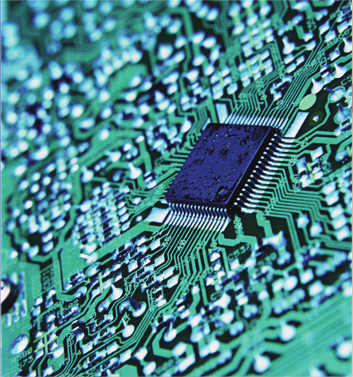Year Name of circuit Transistors $$1971$$ $$4004$$ $$2300$$ $$1972$$ $$8008$$ $$3300$$ $$1974$$ $$8080$$ $$6000$$ $$1978$$ $$8086$$ $$29,000$$ $$1979$$ $$8088$$ $$30,000$$ $$1982$$ $$80286$$ $$134,000$$ $$1985$$ $$80386$$ $$275,000$$ $$1989$$ $$90486$$ $$1,200,000$$ $$1993$$ Pentium $$3,000,000$$ $$1995$$ Pentium Pro $$5,500,000$$ $$1997$$ Pentium II $$7,500,000$$ $$1998$$ Pentium II Xeon $$7,500,000$$ $$1999$$ Pentium III $$9,500,000$$The data shown are modeled by the exponential function $$N(t) = 2200(1.356)^t\text{,}$$ where $$t$$ is the number of years since $$1970\text{.}$$